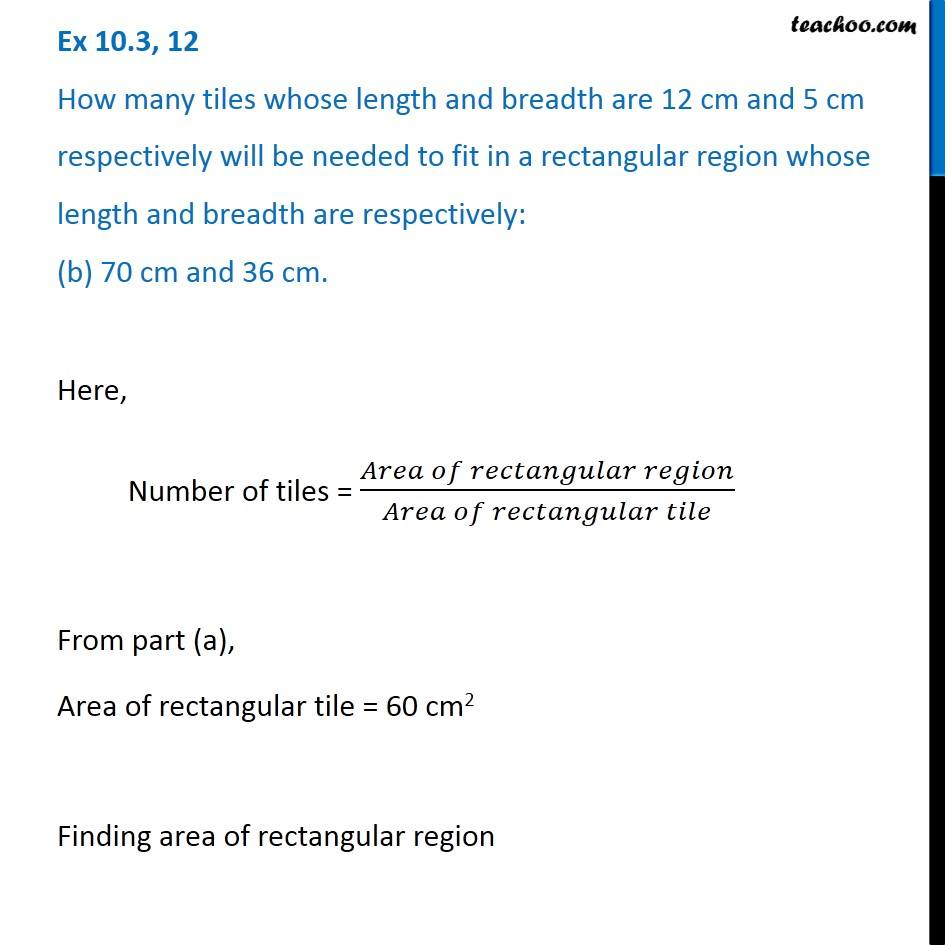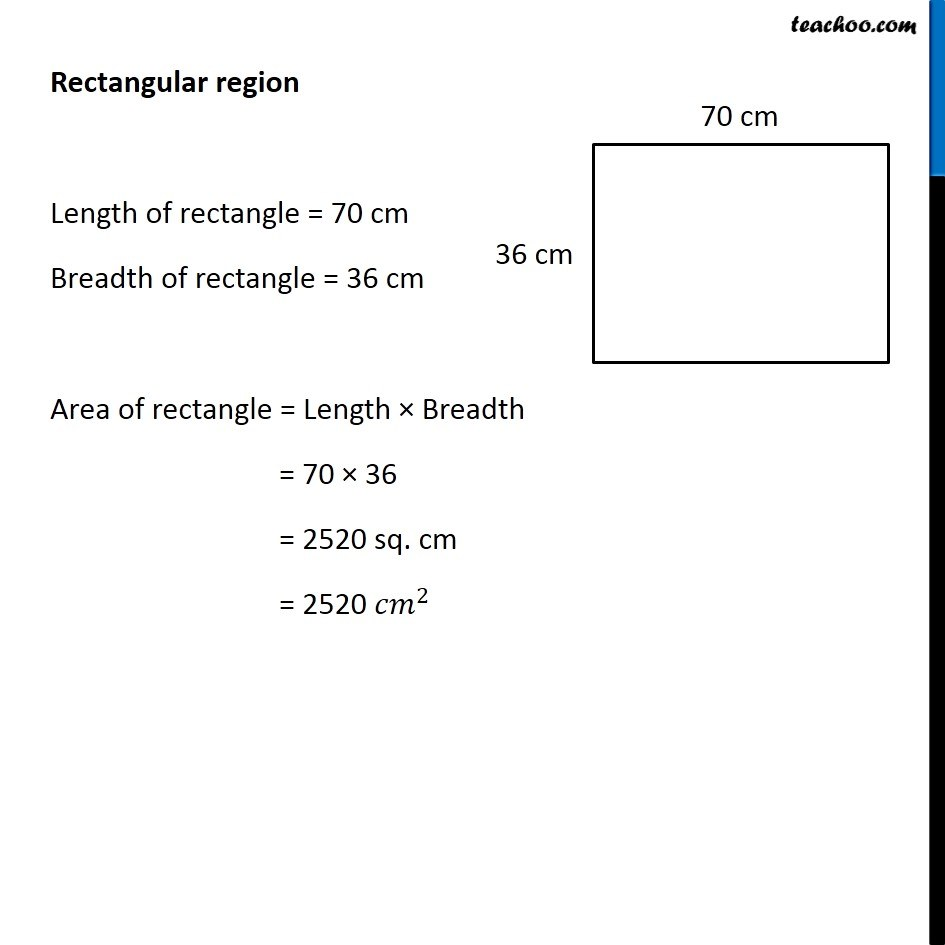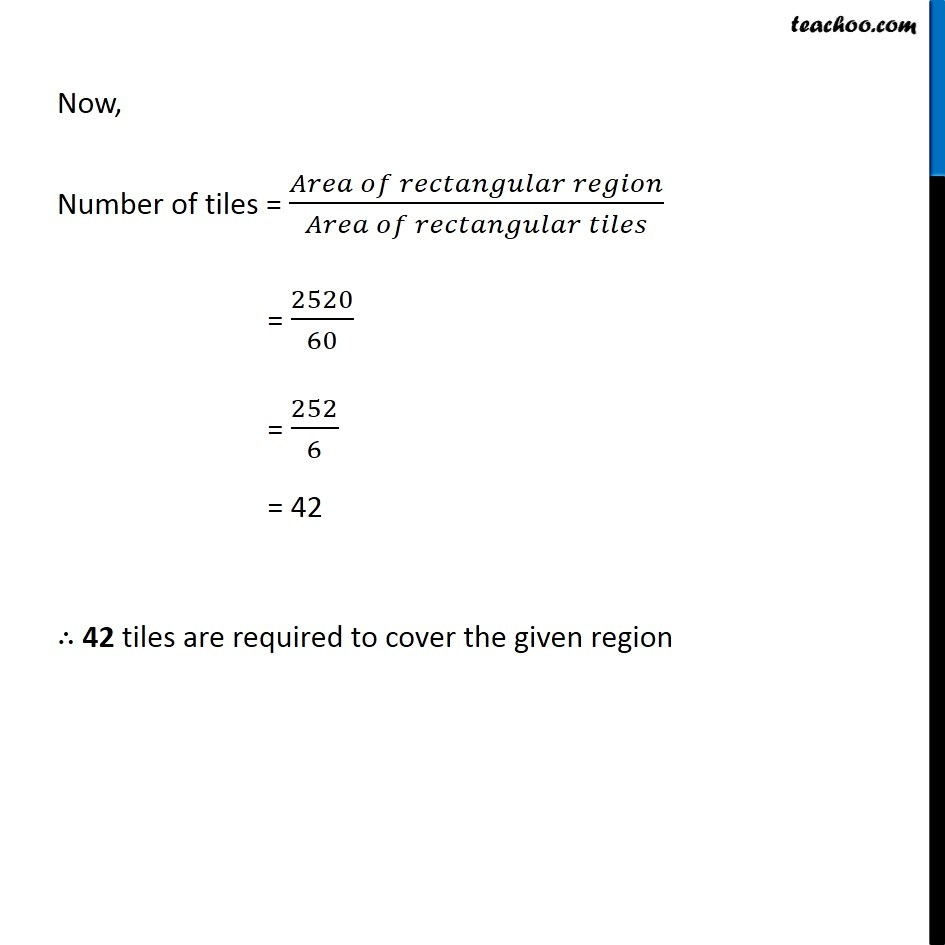Ex 10.3

Chapter 10 Class 6 Mensuration
Serial order wiseGet live Maths 1-on-1 Classs - Class 6 to 12

### Transcript

Ex 10.3, 12 How many tiles whose length and breadth are 12 cm and 5 cm respectively will be needed to fit in a rectangular region whose length and breadth are respectively: (b) 70 cm and 36 cm. Here, Number of tiles = (𝐴𝑟𝑒𝑎 𝑜𝑓 𝑟𝑒𝑐𝑡𝑎𝑛𝑔𝑢𝑙𝑎𝑟 𝑟𝑒𝑔𝑖𝑜𝑛)/(𝐴𝑟𝑒𝑎 𝑜𝑓 𝑟𝑒𝑐𝑡𝑎𝑛𝑔𝑢𝑙𝑎𝑟 𝑡𝑖𝑙𝑒) From part (a), Area of rectangular tile = 60 cm2 Finding area of rectangular region Rectangular region Length of rectangle = 70 cm Breadth of rectangle = 36 cm Area of rectangle = Length × Breadth = 70 × 36 = 2520 sq. cm = 2520 〖𝑐𝑚〗^2 Now, Number of tiles = (𝐴𝑟𝑒𝑎 𝑜𝑓 𝑟𝑒𝑐𝑡𝑎𝑛𝑔𝑢𝑙𝑎𝑟 𝑟𝑒𝑔𝑖𝑜𝑛)/(𝐴𝑟𝑒𝑎 𝑜𝑓 𝑟𝑒𝑐𝑡𝑎𝑛𝑔𝑢𝑙𝑎𝑟 𝑡𝑖𝑙𝑒𝑠) = 2520/60 = 252/6 = 42 ∴ 42 tiles are required to cover the given region